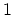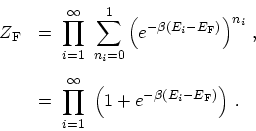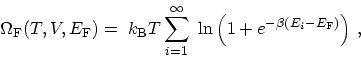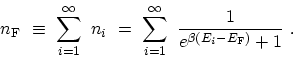# C.1 FERMI-DIRAC Statistics

For FERMIons the occupation numbers are either 0 or, and the sum in (C.12) is restricted to these values(C.13)

Taking the logarithm of both sides, one gets(C.14)

while the mean number of FERMIons is given by the FERMI-DIRAC distribution function(C.15)

M. Pourfath: Numerical Study of Quantum Transport in Carbon Nanotube-Based Transistors